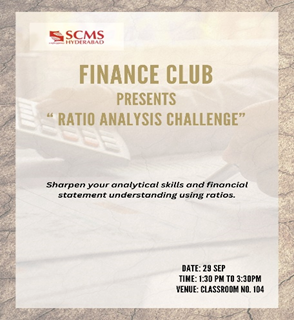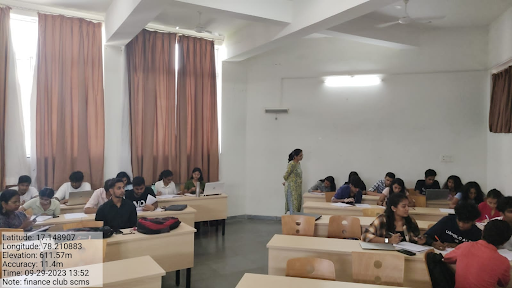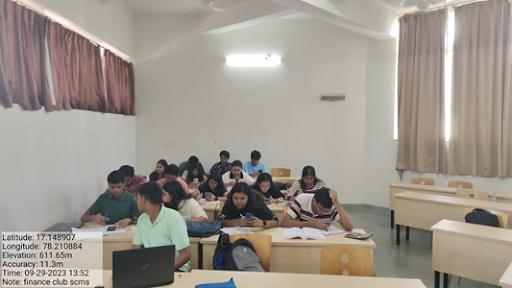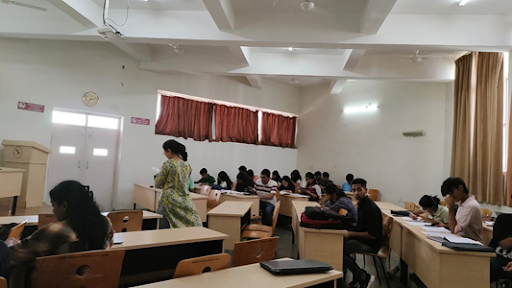# Ratio Analysis Challenge

#### Summary

Event Name: Ratio Analysis Challenge

Objectives : To enhance students' understanding of financial concepts & familiarize the students with the importance and uses of ratios analysis.

Outcome :

• At the end of the activity the students gained knowledge about how to calculate ratios, analyze the ratios and give relevant interpretations.
• The activity succeeded in engaging the students, making them more eager to explore the subject further.Brief Report :

The first activity of Finance club was kicked off by our respected in-charge of Finance club, Dr. Disha Pathak, who first announced the procedure and rules of the activity. As planned, we had 8 teams consist of five students in each team. Each team was assigned one of NIFTY50 company’s balance sheet and profit & loss statement. Each team was required to calculate and interpret below mentioned ratios for the given data of two years in an hour time:

1. Liquidity ratio
• Current ratio
• Cash ratio
2. Leverage ratio
• Debt ratio
• Interest coverage ratio
3. Efficiency ratio
• Asset turnover ratio
4. Profitability ratio
• Return on asset ratio
• Net profit ratio
• Operating profit ratio
5. Market value ratio
• Earnings per share
• Price earnings ratio(Dr. Disha Pathak guiding the students)

In a team, students calculated the ratios and gave their interpretation comparing the ratios of two years. Also, the faculty member asked the questions to each team members for checking their understanding.
In conclusion, this event garnered an amazing participation from our batchmates.(Students working on the challenge)